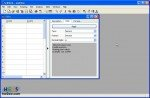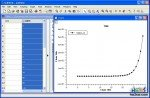Skip to content

# SciDAVis. Art of approximationSciDAVis starts with two windows, whose one is a table where to add data, so you know by first impact what this application requires to work.

For people who isn't familiar with this kind of software any job could appear without any sense; that's why i prepared a project file and a txt with numbers representing the Fibonacci series up to 30.

Fibonacci's is a recursive series working this way:

• F(0)=0, that's the first basic value or step to let the series start;
• F(1)=1, second basic value;
• F(n)=F(n-1)+F(n-2), where n is an integer positive number.

Well... F(2)=?
F(2)=F(1)+F(0)=1+0=1;
F(3)=F(2)+F(1)=1+1=2;
F(4)=F(3)+F(2)=2+1=3; and so on.

With SciDAVis installed, open zip file you downloaded and doubleclick to fibonacci.sciprj which will open a filled table, or open run SciDAVis and by File menu choose "Import ASCII". You're done!

To become a little bit comfortable with it begin playing with some commands and functions; plotting could be very interesting because the graph gives you the behavior, especially when you have a big quantity of numbers which to work with.For example you can plot loaded series following two steps:

1. choose the column to plot. On table's window click on C2[Y] voice on top;
2. go to Plot menu -> "Special Line/Symbol" -> Spline.

You'll get same as on right picture.

Adding a third column 3D plotting becomes available.You can analyze many format pictures (not jpeg) by "Import Image..." voice in File menu: a matrix will be created, not a simple table, with detailed informations about picture.
For example our hw2sw logo returns a matrix (partially visible on left), that 3D-plotted gives the right graph you can rotate and modify even only by colors.
It's possible to export the graph as image directly by right-clicking on it -> Export or from File Menu -> Export Graph.